# Excel VBA Tutorial: Understanding the difference between Range and Cells in Excel VBA

Last updated on June 29, 2018 By

Summary: Learn the difference between using Range() and Cells() when referring to ranges with Excel VBA. With the help of sample code you’ll understand when to use Range() and when to use Cells(), how to use them together, and also get bonus tips for working with ranges.

Difficulty: Beginner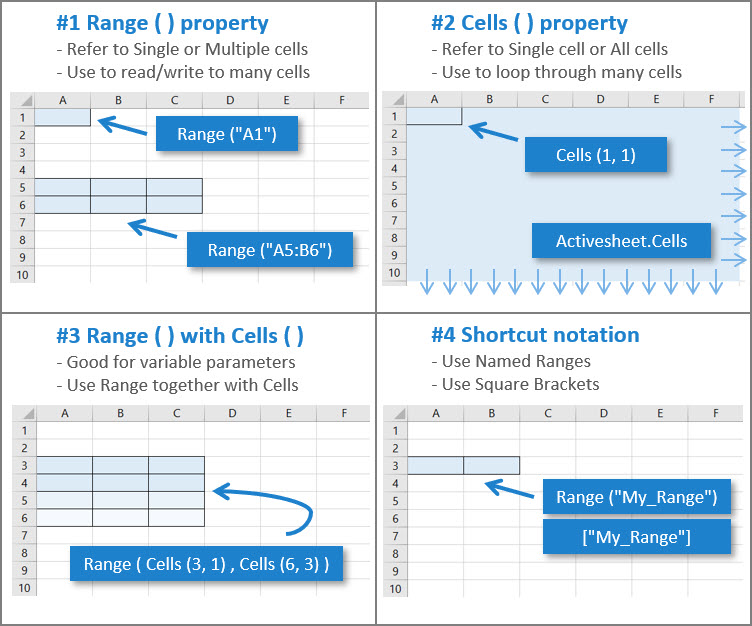Introduction to Ranges in VBA

It’s great to learn how to properly refer to ranges in Excel VBA. They are the bread and butter of Excel. After all, pretty much every workbook you use contains data in cells that you want to manipulate!

But… beginners are often confused because there are different ways to refer to ranges.

Range() and Cells() are two common ways to refer to ranges in Excel VBA. And they do pretty similar things, which is where the confusion starts.

So let me help clear up any confusion you might have…

Excel VBA Cheat Sheets » Find it hard to write Excel VBA Macros? Think there’s too much to remember? You’re not alone! Check out my convenient one-page guides. They’ll help you write Excel VBA Macros better and faster.

## #1 – Range – refers to Single Cells or Multiple Cells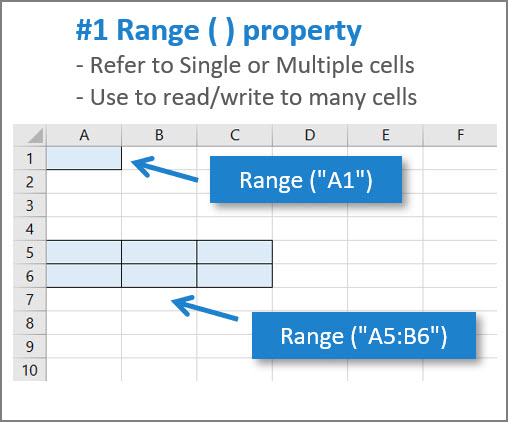## Where Range fits into the Object Model

Let’s have a look at where the Range property fits in the Excel Object Hierarchy (this is basically the language structure and how you access things in VBA):

Excel Application > Workbook > Worksheet > Range

Every worksheet in Excel has a Range property in VBA.

You use this to refer to cells on the worksheet.

It works for single cells and multiple cells.

There’s two ways to use Range:

1. With one argument – like Range(“A1:C3”)
2. With two arguments – like Range(“A1”, “C3”)

Don’t worry if this theory doesn’t make 100% sense right now…

Let’s look at some VBA code samples to see how it works in practice.

## Method 1 – use Range with one argument

The Range property uses cell references just like most Excel functions (e.g. “A1” or “A1:C20”)

It’s easy for most Excel users to learn Range because it looks familiar.

The argument goes inside double quotes.

Here are some examples:

```Sub Range_Examples_1()
' PURPOSE : Show ways to use Range property

'Write values to the Active Sheet
Range("A1") = 1
Range("A1:C3") = 2
Range("A3, B2, C1") = 3

End Sub
```

All of these use Range() with one argument. When using Range() with one argument, it must be an address string (which can include a workbook and/or worksheet name) or the name of a range.

And all the above refer to ranges on the Active Sheet. When you don’t specify a worksheet, Excel uses the Active Sheet in the Current Workbook.

Notice you can refer to multiple cells using a colon ( : ) like “A1:C3” or using a comma ( , ) like “A3, B2, C1”.

Just remember to put the range reference inside double quotes.

Here are some examples where the worksheet is specified:

```Sub Range_Examples_2()
' PURPOSE : Show ways to use Range property

'Using Worksheet Name in the argument
Range("Sheet1!A1") = 1

'Using Worksheet Name to specify worksheet object
Worksheets("Sheet1").Range("A1") = 1

'Using Code Name to specify worksheet object
Sheet1.Range("A1") = 1

End Sub
```

When you specify a worksheet, Excel uses that worksheet instead of the Active Sheet.

In case you’re not familiar, there are two ways to refer to worksheets.

1. Worksheet name – This is what the user sees in the normal Excel interface.
2. Code name – This is an internal name used when writing VBA.

Here’s a diagram that shows where you can find these different names in the VBA Editor: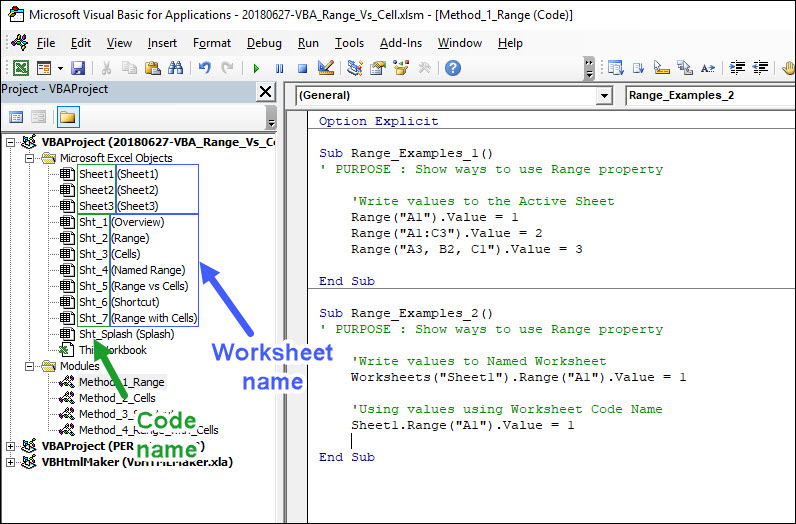## Method 2 – Range with two arguments

To use Range() with two arguments you separate them with a comma ( , ) like this:

```Sub Range_Examples_3()
' PURPOSE : Show ways to use Range property

'Use two arguments that are address strings
Range("A1", "C3") = 1

'Use two arguments with one being Active Cell
Range("B6", ActiveCell) = 2

End Sub
```

This image shows what ranges the VBA code refers to: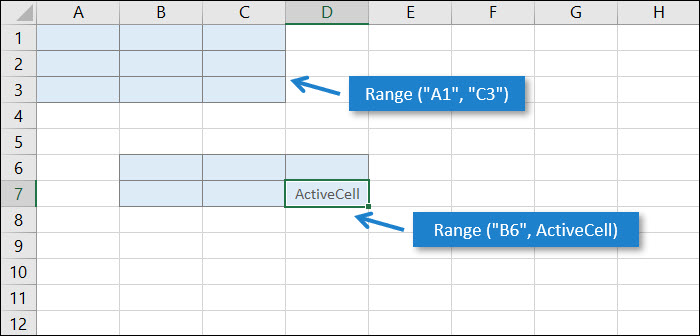When you use Range() with two arguments, the arguments can be Range objects, address strings (but cannot include workbook/worksheet names), or the names of ranges, or a combination of these.

The following shows examples of valid and invalid code:

```Sub Range_Examples_4()
' PURPOSE : Show ways to use Range property

'These are valid ways to use two arguments
Range("A1", "E5") = 1
Range("A1", Range("E5")) = 1
Range(Range("A1"), Range("E5")) = 1
Range("Named_Range_1", "Named_Range_2") = 1

'This is NOT a valid way to use two arguments
Range("Sheet1!A1", "Sheet1!C3") = 1

End Sub
```

## #2 – Cells – refers to Single cells or All cells

Every worksheet in Excel has a Cells property in VBA.

You use Cells() to refer to Single cells on the worksheet, or All cells on the worksheet.

The differences between Range and Cells:

• Cells refers to Single cells only – it can’t refer to multiple cells like Range (“A1:E5”)
• Cells takes Row and Column as arguments

Note: Cells can also be used to refer to All cells in a worksheet when written like this: ActiveSheet.Cells or Sheet1.Cells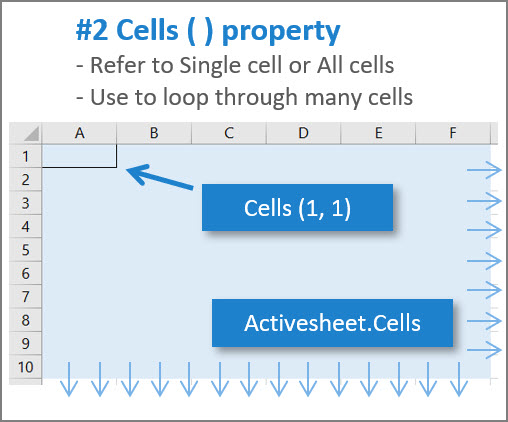The Cells property uses Row and Column references like this – Cells ( Row , Column ) – to locate a cell.

Here are some examples:

```Sub Cells_Examples_1()
' PURPOSE : Show ways to use Cells property

'Select range A1 of Active Sheet
Cells(1, 1).Select

'Select range A5 of Active Sheet
Cells(5, 1).Select

'Select range E1 of Active Sheet
Cells(1, 5).Select

'Select range E5 of Active Sheet
Cells(5, 5).Select

End Sub
```

Remember if you don’t specify a worksheet (by name or code name), Excel assumes you mean the ActiveSheet.

This image shows what ranges the VBA code refer to: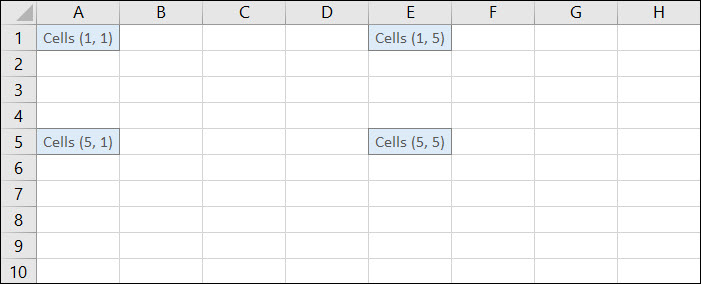## Using letters instead of numbers

When you use Cells (row, column) you must use numbers to specify the Row, but you can use either numbers or letters to specify the Column.

Here’s an example:

```Sub Cells_Examples_2()
' PURPOSE : Show ways to use Cells property

'Using numbers
Cells(5, 5).Select

'Using number and letter
Cells(5, "E").Select

'Note: for the Row you must use numbers
'Note: for the Column you can use either numbers or letters

End Sub
```

## Using Cells to refer to All Cells

If you don’t specify row and column, Excel will refer to all cells on the worksheet.

Take this example, which clears the contents of all cells on the Active Sheet.

```Sub Cells_Examples_3()
' PURPOSE : Show ways to use Cells property

'Clear contents of all cells on Active Sheet
ActiveSheet.Cells.ClearContents

End Sub
```

## Use Cells() for looping – a great reason to use Cells()

Cells() is great for looping through rows or columns because you can specify the row / column using numbers.

Here’s a simple example that populates “A1:E5” with the product of row and column (= Row x Column) in double nested For loops.

```Sub Cells_Examples_4()
' PURPOSE : Show ways to use Cells property

'Fill cells with product of row and column
Dim R As Integer
Dim C As Integer

For C = 1 To 5
For R = 1 To 5
Cells(R, C) = R * C
Next R
Next C

End Sub
```

This image shows what happens when you run the VBA code: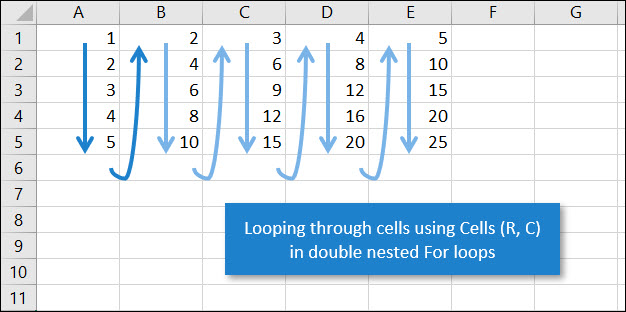## #3 – Range with Cells – use Range() together with Cells() to define start and end of a range with variables

You can combine Range with Cells .

You write it like this – Range ( Cells (3, 1), Cells (6, 3) )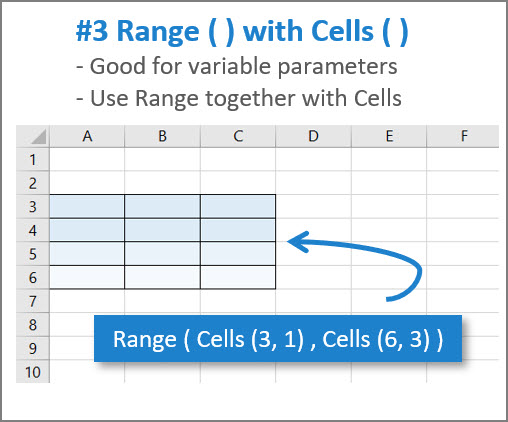This is really useful when you want to have variables to specify your range.

For example you can replace the numbers with variables like this – Range ( Cells (3, 1), Cells (Last_Row, Last_Column) ) – where Last_Row and Last_Column are VBA variables.

Check out this VBA code for a simple use case:

```Sub Range_With_Cells()
' PURPOSE : Show ways to use Range together with Cells

Dim Red As Integer
Dim Green As Integer
Dim Blue As Integer
Dim R As Integer

Red = 140
Green = 200
Blue = 230

'Format rows 2 to 6 in different hues of blue
For R = 2 To 6
Range(Cells(R, 2), Cells(R, 4)) _
.Interior.Color = RGB(Red, Green, Blue)
Red = Red + 20
Green = Green + 10
Blue = Blue + 5
Next R

End Sub
```

This is better than using Cells in a nested For loop because you don’t need to loop through every single cell. Instead you are looping through whole ranges of cells, row by row, from row 2 to row 6.

This image shows what happens when you run the code: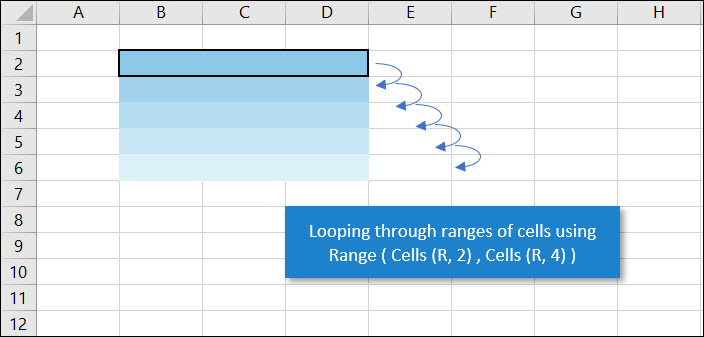## #4 – Shortcut Notation – with named ranges and square brackets

You can refer to ranges with a couple of shortcuts: Named Ranges and Square Brackets.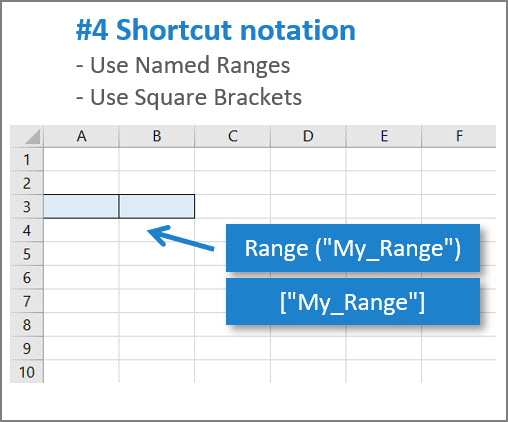### Named Ranges

Named Ranges are useful to specify a range using a typed name – like “My_Range” – that can be defined in the Excel interface using the Name Manager (CTRL + F3) or defined in VBA.

Named Ranges can stay the same in your VBA code, while you change the actual range in the Excel interface or in another part of VBA code.

This means you don’t need to hard code a range – like Range (“A1 : E25”) – when you could use a Named Range instead – like Range (“My_Data” ).

### Square Brackets [ ]

Use square brackets like this [A1] and [A1 : E25] as a shortcut.

When you use square brackets you are actually calling the Evaluate method with a string argument. For further reading see: Application.Evaluate Method (Excel) on Microsoft’s Dev Center website.

But…from what I hear, this method isn’t very widespread and it might confuse people reading your code if you use this shortcut.

So use square brackets with this in mind.

Here is a table comparing some different ways of referring to ranges: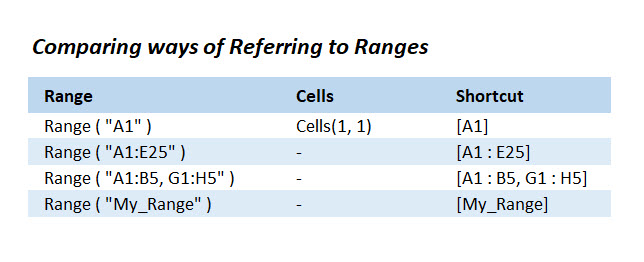## #5 – Range vs Cells – when is it better to use Range? And when use Cells?

### When to use Range

When there’s an operation that needs to be done over a range in one go (such as range formatting or filling a range with the same formula), it’s better to use Range instead of Cells.

```Sub Range_Examples_5()
' PURPOSE : Show ways to use Range property

'Formatting a range
Range("A1:E5").Interior.Color = RGB(180, 180, 180)

End Sub
```

### When to use Cells

When there’s an operation that needs looping through individual cells, It’s better to use Cells(row,column), because It’s easier to keep counters for both row and column.

Like in this example from before:

```Sub Cells_Examples_4()
' PURPOSE : Show ways to use Cells property

'Fill cells with product of row and column
Dim R As Integer
Dim C As Integer

For R = 1 To 5
For C = 1 To 5
Cells(R, C) = R * C
Next C
Next R

End Sub
```

## #6 – BONUS Tips – for working with ranges – avoid these rookie mistakes!

Here are three bonus tips for you to keep in mind. Avoid these rookie mistakes to improve your VBA.

1. Avoid Selecting ranges – Your VBA code doesn’t need to select a range to work with it. When you use the Macro Recorder it generates code that selects ranges. In your VBA code you seldom need to do this (and in fact it slows the code down significantly).
2. If you must SelectYou can’t select a range that’s not on the active worksheet. So if you need to select a range, the worksheet it’s on must be active. You can use the Activate method on the worksheet to do this.
3. Specify worksheet objects – It’s good practice to specify a worksheet object for every range you work with (unless you are sure you want to operate on the Active Sheet whatever that is). If you don’t specify the worksheet, your code might work today but fail another day if the ActiveSheet is different from what you assume.

Bonus Tip 3 needs more explanation because it’s surprisingly easy to make a mistake when specifying worksheets when you combine Range and Cells.

Take a look at this code:

```Sub Bonus_Tip_Explained()
' PURPOSE: look at referencing worksheets

'This is wrong
Worksheets("Sheet1").Range(Cells(1, 1), Cells(5, 5)) = 1

End Sub
```

Why is this wrong?

First, notice that the Range property is qualified. It belongs to Worksheets(“Sheet1”) and you can tell this because there’s a dot between Worksheets and Range.

Second, notice that the Cells properties are not qualified. They belong to the ActiveSheet and not Worksheets(“Sheet1”).

So this code works sometimes and fails at other times.

If the code is in a code module, then it works when the ActiveSheet happens to be Sheet1. But it fails when the ActiveSheet is not Sheet1.

If the code is in a worksheet code module, then any use of Range or Cells without a worksheet object reference refers to the worksheet containing the code and not the ActiveSheet. So it works if inside the Sheet1 code module, but doesn’t work if in another worksheet code module.

Here’s an image showing which part is wrong, and also how to correct it: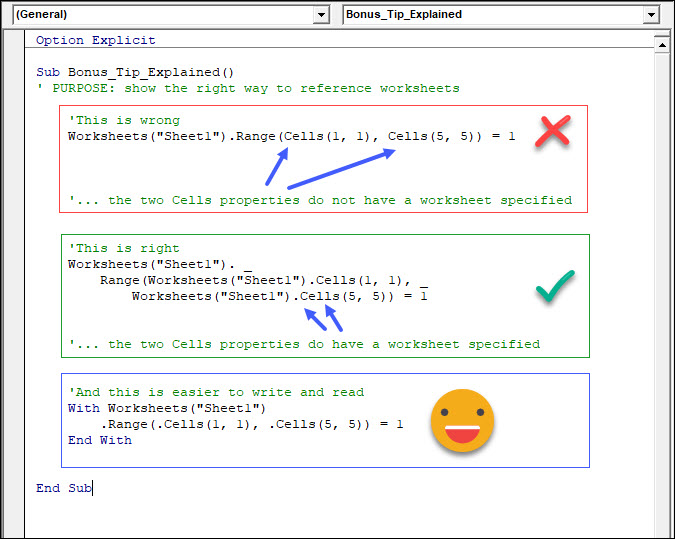For your copy/paste convenience… here’s the VBA code for the With … End statement:

```    With Worksheets("Sheet1")
.Range(.Cells(1, 1), .Cells(5, 5)) = 1
End With
```

## #7 – Recap – remember what you learned

OK let’s do review of the main points. And if you’re serious about learning this material, I strongly recommend you test your recall now by doing the exercises in Excel. Remember to have fun too!

### Range

• Use the Range property to specify a single cell or multiple cells.
• Use it with one argument – e.g. Range (“A1”) or Range(“A1:C3”).
• Use it with two arguments – e.g. Range(“A1”, “C3”) or Range(“A1”, ActiveCell).
• If you don’t specify a worksheet Excel will use the Active Sheet.
• Exercise: use the Range property to fill the range A1:C10 of the Active Sheet with the value 5.

### Cells

• Use the Cells property to specify a single cell or all cells on the worksheet.
• Use it with two arguments – e.g. Cells(1, 5) for row 1 and column 5 OR Cells(1, “E”) for row 1 and column E.
• Use it with no arguments – e.g. ActiveSheet.Cells for all cells on the Active Sheet.
• If you don’t specify a worksheet Excel will use the Active Sheet.
• Exercise: use the Cells property to fill range A1:E5 with the sum of row and column in each cell. (Hint: we saw some code that filled the product of row and column in each cell)

### Range with Cells

• Combine Range with Cells – e.g. Range(Cells(3, 1), Cells(6, 3))
• Useful when you want to use variables to specify your range.
• Example: Use variable R to loop through values 1 to 10 using Range(Cells(R, 2), Cells(R, 4)), which loops through rows 1 to 10 of columns B:D.
• Exercise: use Range with Cells to fill range B1:D10 with the row number.

### Shortcut Notation

• Refer to named ranges – e.g. Range(“My_Range”).
• Use square brackets – e.g. [A1:E25] which is the same as Range(“A1:E25”)
• Exercise: use square brackets to fill range A1:C10 of the Active Sheet with the value 5.

### Range vs Cells

• Use Range when there’s an operation that needs to be done over a range in one go.
• Exercise: use Range to format range A1:E5 of the Active Sheet with the background color RGB(190, 190, 190) (Hint: we looked at code above that does something very similar)
• Use Cells when there’s an operation that needs looping through individual cells.
• Exercise: use Cells to format range A1:P16 (16 rows, 16 columns) of the Active Sheet with the background color RGB(row x column, row x column, row x column)

### BONUS Tips

• Avoid Selecting ranges – instead change the value or other property directly
• If you must Select… – you can only select a range that’s on the Active Sheet
• Specify worksheets – it’s a good idea to always specify the worksheet for each range you use

Here are references you might find useful.

I wrote this article to be comprehensive… but I didn’t want it to turn into a huge reference manual! So here are some references in case you want to learn even more about Ranges and Cells.

Excel VBA Cheat Sheets » Find it hard to write Excel VBA Macros? Think there’s too much to remember? You’re not alone! Check out my convenient one-page guides. They’ll help you write Excel VBA Macros better and faster.

### Related Posts:Author: Victor Chan

Victor expertly teaches Microsoft Excel to people all over the world. He has millions of views of his popular Excel explainer videos on YouTube. These show time-saving shortcuts and real-world applications explained with easy-to-follow visuals.

Victor has over 20 years of experience using Excel as a professional for Big 4 Audit Firm Deloitte and two global tech companies. He knows firsthand that being more productive with Excel can lead to greater job satisfaction and career growth.

Recommended Posts

Discover the PROVEN Blueprint for transforming your Excel skills, supercharging your productivity, and standing out in your career! My course helps you to learn Excel VBA and save hours of time even if you have zero prior experience with programming.

Solve tricky Excel problems and take your work to the next level! Get customized solutions for your unique needs. Save time and gain insights with truly expert Excel solutions from only \$97 per task.

Get a clear overview of your project progress using the Excel project timeline. Use it to communicate the big picture, track task progress, and stay on top of your project goals. Stay organized with our project timeline!

Our cheat sheets provide quick and easy reference to commonly used Excel VBA concepts and code snippets.

Unlock new levels of productivity and efficiency with our cheat sheets, and write VBA code like a pro in no time.